Try out our new practice tests completely free!# Quiz 2: Diodes and Applications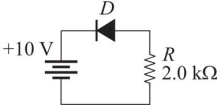Figure 4 -Refer to Figure 4. Assume the diode is a practical diode. If the diode is reversed, the current in the diode will be(Multiple Choice)

ATo convert the rms value of a sine wave to a peak value,(Multiple Choice)

BA voltage regulator compensates for changes in(Multiple Choice)

D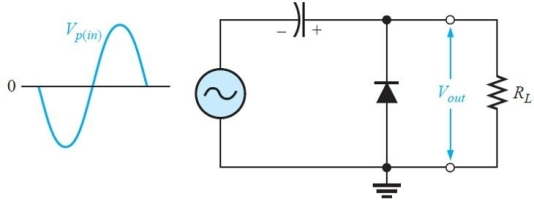Figure 8 -The maximum output voltage for the circuit in Figure 8 is approximately (Assume an ideal diode.)
(Multiple Choice)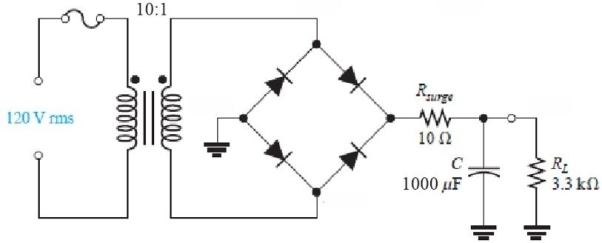Figure 5 -Refer to the circuit in Figure 5. The rms primary current is approximately
(Multiple Choice)Reverse current in a diode increases if
(Multiple Choice)The ripple frequency of a bridge rectifier is
(Multiple Choice)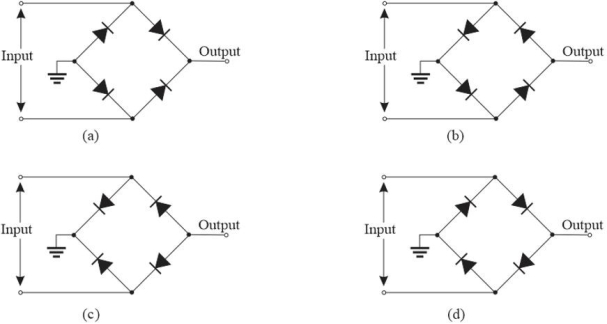Figure 2 -Refer to Figure 2. The circuit that will produce a positive output is
(Multiple Choice)The ideal dc output voltage of a capacitor- input filter equals the
(Multiple Choice)Another name for a diode limiter circuit is
(Multiple Choice)A capacitor filtered half- wave rectifier has a ripple frequency that is
(Multiple Choice)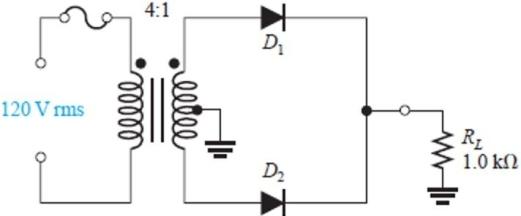Figure 1 -The circuit in Figure 1 is a
(Multiple Choice)Figure 5 -Refer to the circuit in Figure 5. The purpose of Rsurge is to
(Multiple Choice)Assume an ideal diode has a series 10 k▲ resistor and is reverse- biased with a 5.0 V source. The current in the diode is
(Multiple Choice)The VRRM specification on a manufacturer's data sheet is the same as the
(Multiple Choice)In a full wave bridge rectifier with a capacitor filter, assume a diode has opened. You expect to see
(Multiple Choice)Figure 8 -The circuit in Figure 8 is a
(Multiple Choice)The forward resistance of a diode is
(Multiple Choice)C:\User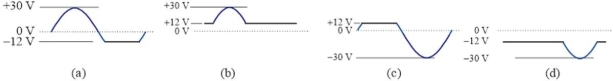Figure 7 For questions 34, 35, and 36, assume ideal diodes -Which circuit in Figure 6 will produce the waveform in Figure 7(c)?
(Multiple Choice)A capacitor filtered full- wave rectifier has a ripple frequency that is
(Multiple Choice)## Filters

• Essay(0)
• Multiple Choice(0)